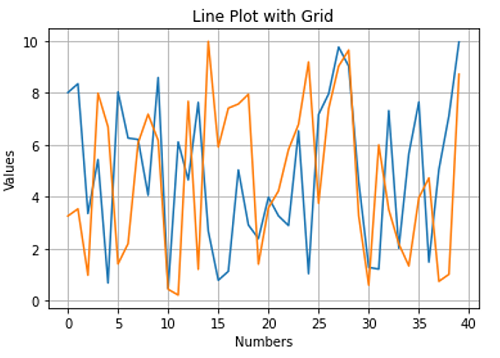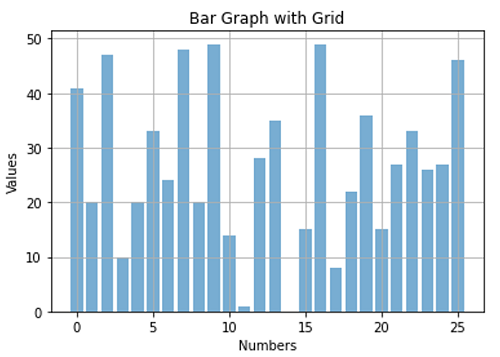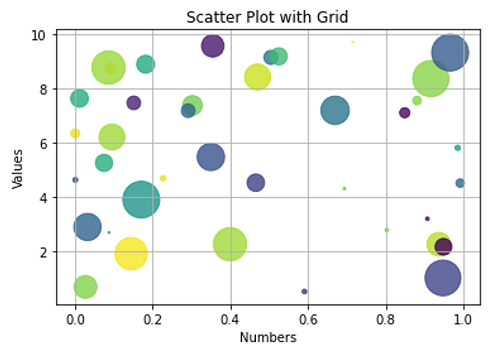# Python | Grid to the plot

Python | Grid to the plot: In this tutorial, we are going to learn about the grid to the plot and its implementation with examples.
Submitted by Anuj Singh, on July 09, 2020

Most of the time, we need good accuracy in data visualization and a normal plot can be ambiguous. So, it is better to use a grid that allows us to locate the approximate value near the points in the plot. It helps in reducing the ambiguity and therefore, there is a function plt.grid() which generates a grid through the plot and enables better visualization.

The following are examples for understanding the implementation Grid.

## 1) Line plot with Grid## 2) Bar Graph with Grid## 3) Scatter Plot with Grid## Python code to demonstrate example of grid to the plot

```# Data Visualization using Python

import numpy as np
import matplotlib.pyplot as plt

# Line Plot
N = 40
x = np.arange(N)
y = np.random.rand(N)*10
yy = np.random.rand(N)*10
plt.figure()
plt.plot(x,y)
plt.plot(x,yy)
plt.xlabel('Numbers')
plt.ylabel('Values')
plt.title('Line Plot with Grid')
plt.grid()
plt.show()

# Bar Graph
N = 8
x = np.array([1,2,3,4,5,6,7,9])
xx = np.array(['a','b','c','d','e','f','g','u'])
y = np.random.rand(N)*10
plt.figure()
plt.bar(np.arange(26), np.random.randint(0,50,26), alpha = 0.6)
plt.xlabel('Numbers')
plt.ylabel('Values')
plt.title('Bar Graph with Grid')
plt.grid()
plt.show()

# Scatter Plot
N = 40
x = np.random.rand(N)
y = np.random.rand(N)*10
colors = np.random.rand(N)
area = (30 * np.random.rand(N))**2  # 0 to 15 point radii
plt.figure()
plt.scatter(x, y, s=area, c=colors, alpha=0.8)
plt.xlabel('Numbers')
plt.ylabel('Values')
plt.title('Scatter Plot with Grid')
plt.grid()
plt.show()
```

Languages: » C » C++ » C++ STL » Java » Data Structure » C#.Net » Android » Kotlin » SQL
Web Technologies: » PHP » Python » JavaScript » CSS » Ajax » Node.js » Web programming/HTML
Solved programs: » C » C++ » DS » Java » C#
Aptitude que. & ans.: » C » C++ » Java » DBMS
Interview que. & ans.: » C » Embedded C » Java » SEO » HR
CS Subjects: » CS Basics » O.S. » Networks » DBMS » Embedded Systems » Cloud Computing
» Machine learning » CS Organizations » Linux » DOS
More: » Articles » Puzzles » News/Updates